﻿ Large Data Analysis via Interpolation of Functions: Interpolating Polynomials vs Artificial Neural NetworksAmerican Journal of Intelligent Systems

p-ISSN: 2165-8978    e-ISSN: 2165-8994

2018;  8(1): 6-11

doi:10.5923/j.ajis.20180801.02### Large Data Analysis via Interpolation of Functions: Interpolating Polynomials vs Artificial Neural Networks

Rohit Raturi

Enterprise Solutions, KPMG LLP, Montvale, USA

Correspondence to: Rohit Raturi, Enterprise Solutions, KPMG LLP, Montvale, USA.
 Email: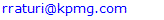Abstract

In this article we study function interpolation problem from interpolating polynomials and artificial neural networks point of view. Function interpolation plays a very important role in many areas of experimental and theoretical sciences. Usual means of function interpolation are interpolation polynomials (Lagrange, Newton, splines, Bezier, etc.). Here we show that a specific strategy of function interpolation realized by means of artificial neural networks is much efficient than, e.g., Lagrange interpolation polynomial.

Keywords: Function interpolation, Approximation, Artificial intelligence, Input layer, Output layer, Hidden layer, Optimization, Weight, Bias, Machine learning

Cite this paper: Rohit Raturi, Large Data Analysis via Interpolation of Functions: Interpolating Polynomials vs Artificial Neural Networks, American Journal of Intelligent Systems, Vol. 8 No. 1, 2018, pp. 6-11. doi: 10.5923/j.ajis.20180801.02.

### 1. Introduction

Interpolation of functions is one of the most desirable methods of mathematical analysis. The main problem of function interpolation can be formulated as follows. It is given a finite set of couples1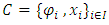where I is a finite set of indexes. The problem is to construct a function,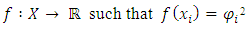Here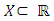is the given interval of the variable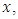on which the interpolation is required. Naturally,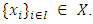Depending on the specific problem under consideration,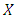can be bounded or unbounded.
Applications of the function interpolation ranges from numerical solution of ordinary and partial differential equations to solution of the best fitting problem for finite sets of data. Usually, real-life observations or measurements at discrete time-instants are gathered in a data set like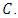For example, in astronomical observations of a planetary motion,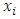are the time-instants when the coordinate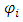of the center of mass of a planet is measured. In bio-laboratory,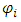maybe the state of a mouse at time-instant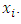The radiation intensity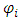is measured at discrete points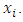Finally, in particle tracing experiments,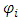may be the coordinate of a particle observed at the time-instant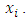In all those examples, it is a very important and challenging problem to know the measuring quantity at all (even non-measured) time-instants. This problem is usually solved by the methods of function interpolation theory [1-3].

### 2. Methods of Interpolation

The theory of function interpolation suggests a variety of methods to tackle such problems. The main tool of function interpolation is the construction of appropriate interpolating polynomials. There exists a handful of interpolating polynomials used in real-life applications. The most known interpolation types are piecewise constant interpolation, linear interpolation, polynomial interpolation, spline interpolation, rational interpolation, Padé approximant, Whittaker-Shannon interpolation (when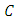is infinite), Hermite interpolation (when both the values of a function and its derivatives are given), wavelets, Gaussian processes (when the interpolating data contain some noise), etc. The choice of a specific interpolating method depends on such criteria as interpolation accuracy, low cost computer realization, ability to cover sparse data sets, etc.
The most delicate analysis require large and sparse data sets. For instance, in the case of large data sets, in order to perform a delicate analysis, the interval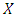must be split into a large number of sub-intervals where the data (observations, measurements, etc.) are easier to interpolate. Then, interpolating functions are interpolated in each sub-interval and eventually the unknown function is represented as the totality of sub-interpolants. In other words, let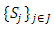be a splitting of the set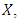i.e,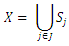and let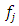be the corresponding interpolants, i.e.,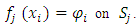Then, the unknown function on the whole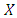is defined as follows: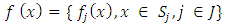Here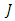is a finite set of indexes. The choice of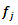is conditioned by the choice of the splitting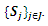If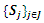contains only several points, then the simple interpolation tools such as piecewise constant or linear interpolation can be used efficiently. Schematic representation of the above mentioned is brought in Fig 1.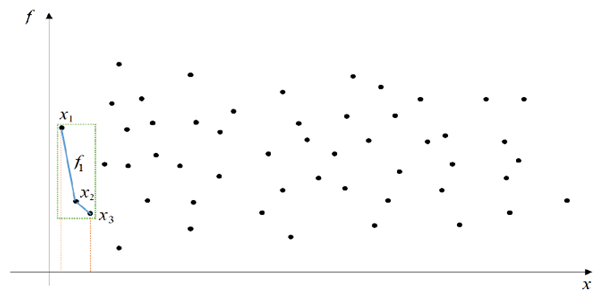Figure 1. Schematic representation of function interpolation over a large data set. Bold points represent the set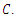Dashed lines draw the boundary of the first splitting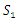consisting of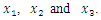Piecewise constant (linear) interpolation is used to construct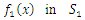. All other interpolating functions are constructed similarly
Remark 1 If the large data set under study is very dense, this approach may lead to enormous computational costs when numerically evaluating the interpolating function f. Contrary, if the large data set under study is sparse, the splitting gives a very efficient strategy. Thus, this approach is valid while the splitting provides a numerically efficient strategy.
Remark 2 Dense data sets are normally formed by the measurements of the very frequent processes or quantitates that changes over coordinate very rapidly. For a greedy algorithm based dense data analysis see, for instance .
Let us now describe some methods of function interpolation. One of the simplest methods of function interpolation is the linear interpolation. This method is valid when the set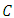consists of only two couples. In such cases, the interpolating function is given as follows: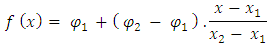Sometimes, piecewise constant (linear) interpolation is combined with the splitting method above to construct a linear interpolating function within each interval of splitting: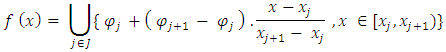In the case of regularly distributed points, polynomial interpolation is usually used. The most common polynomial interpolating functions are:
1. Lagrange interpolating polynomial: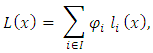Where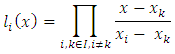2. Newton interpolating polynomial: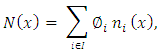Where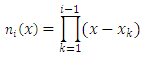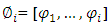is the ith divided difference;
3. Chebyshev interpolating polynomials of the first and second kinds: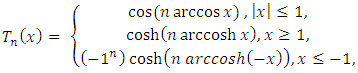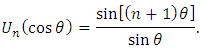In the case of very dense data sets, the spline interpolation is more proffered than polynomial interpolation. As it is well-known, splines are defined piecewise in terms of polynomials of required order: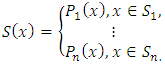Thus, instead of piecewise constant (linear) interpolating functions, splines provide polynomial interpolation within each sub-interval of the splitting.
In the case of periodic or almost periodic data, such as tidal or climate change observation, trigonometric polynomials are used: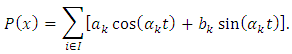### 3. Artificial Neural Networks and Function Interpolation

Artificial neural networks possessing the ability of trained analysis and prediction may be especially advantageous in function interpolation. There exists several algorithms for constructing interpolation of a function by means of artificial neural networks. See, for instance, [5-18] and the related references therein for relevant studies.
The interest towards function interpolation by means of artificial neural networks increased during recent years is conditioned by the advantage shown in the numerical procedure of constructing the interpolating function. Due to the ability of prediction, neural networks allow to consider much less nodes than any other method of interpolation. Thus, solving the same problem requiring a much less computational time and resources. The numerical analysis carried out in the next section shows that it provides a better approximation of two special functions compared with the Lagrange interpolating polynomials with 50 and 100 nodes, respectively.

### 4. Comparison of Lagrange Interpolating Polynomial and Artificial Neural Network Interpolation

In this section we carry out a numerical comparison between interpolation of some well-known specific functions by means of the Lagrange interpolating polynomial and artificial neural networks.
First, let us consider the rectangular function defined according to the piecewise rule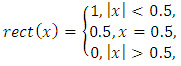Rectangular function has many applications in electrical engineering and signal processing. Besides, it serves as an activation function for many advanced neural networks.
The rect function also can be expressed in terms of the Heaviside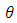function as follows: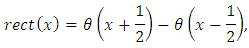Or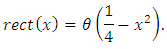Let us remind that the Heaviside function is defined as follows: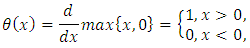Figures 2-4 show the numerical comparison of the interpolation of the rect function by means of Lagrange interpolating polynomial and artificial neural networks. Figure 3 shows that when we consider 50 nodes within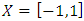, the quadratic error between the Lagrange interpolating polynomial and artificial neural network interpolation dramatically decreases up to 6th epochs. From 7th epoch on, the quadratic error remains almost unaltered and is of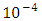order.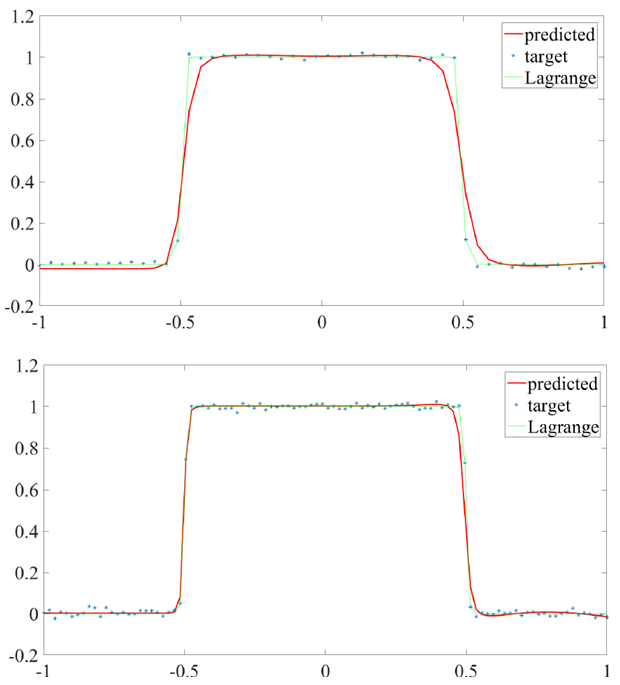Figure 2. Approximation of rect function in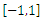50 nodes (upper) and 100 nodes (lower): ANN algorithm requires 10 and 15 iterations, respectively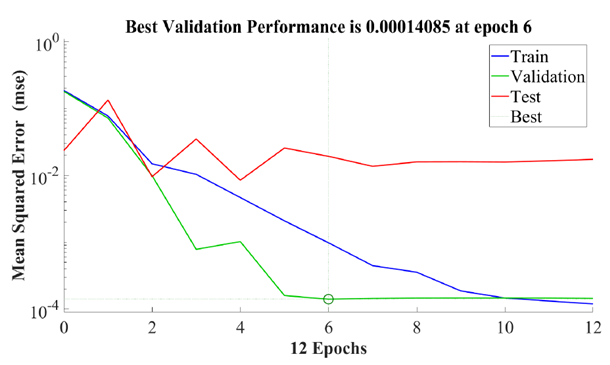Figure 3. Quadratic error approximation of rect function in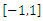with 100 nodes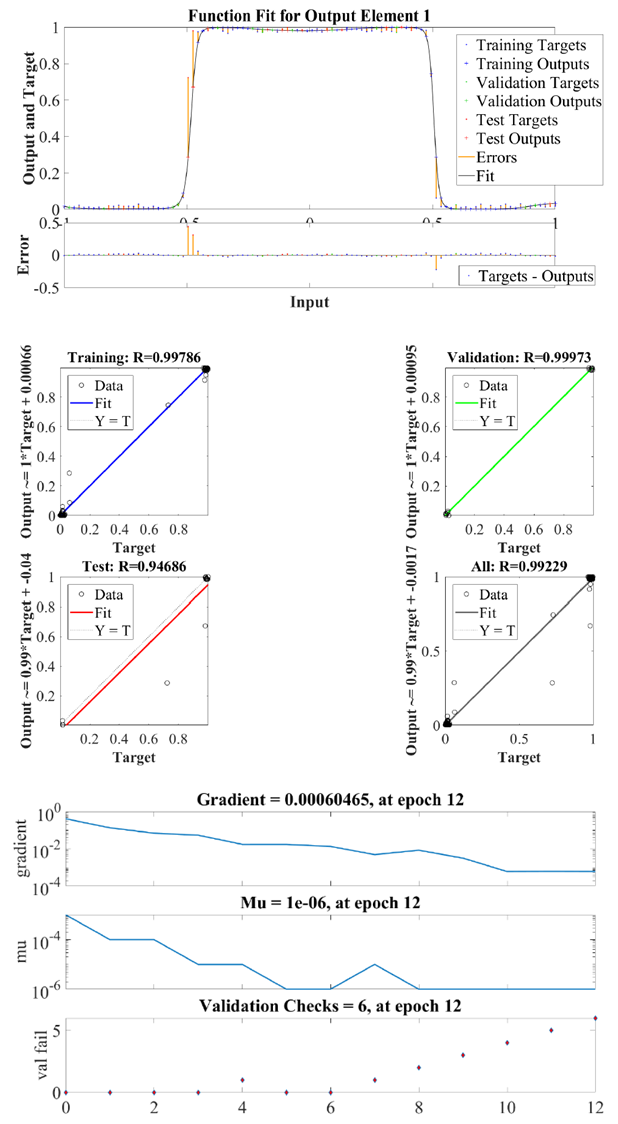Figure 4. Function fit (upper) and regression behaviour (middle) and network performance (lower) for rect function in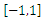with 100 nodes
We also show the advantage of the artificial neural network interpolation in the case of ramp function, defined as follows: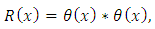where * denotes the convolution operation. Ramp function and has many applications in engineering (it is used in the so-called half-wave rectification, which is used to convert alternating current into direct current by allowing only positive voltages), artificial neural networks (it serves as an activation function), finance, statistics, fluid mechanics, etc.
According to the definition of the Heaviside function, the rump function can be represented also as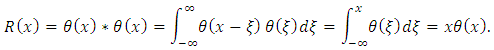The numerical comparison of the interpolation of the ramp function by means of Lagrange interpolating polynomial and artificial neural networks is shown in Figures 5-7. Figure 6 shows that when we consider 50 nodes within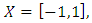the quadratic error between the Lagrange interpolating polynomial and artificial neural network interpolation dramatically decreases up to 5th epochs. From 6th epoch on, the quadratic error remains almost unaltered and is of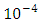order.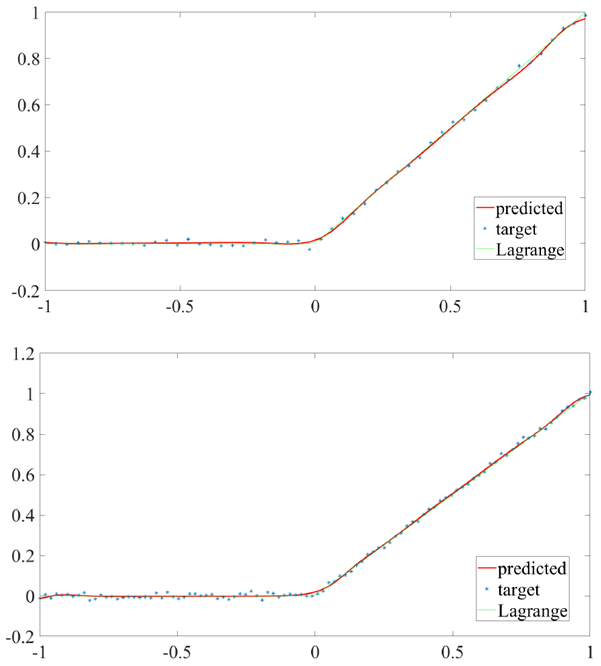Figure 5. Approximation of R function inwith 50 nodes (upper) and 100 nodes (lower): ANN algorithm requires 15 and 29 iterations, respectively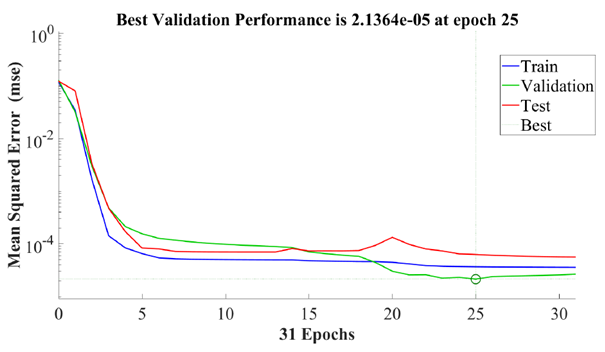Figure 6. Quadratic error approximation of R function in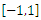with 100 nodes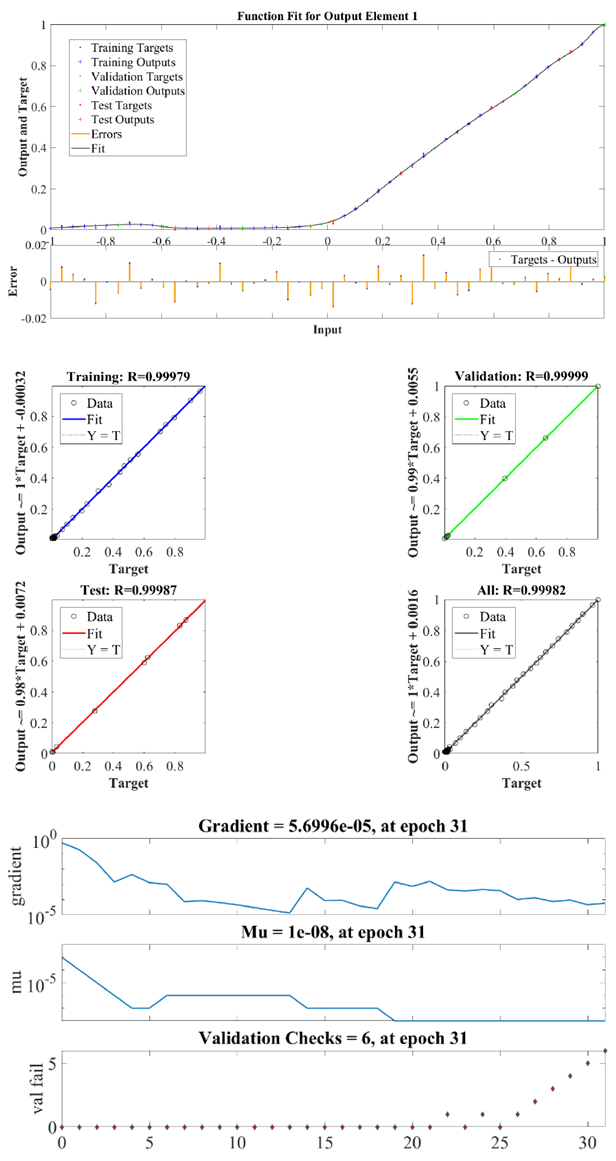Figure 7. Function fit (upper) and regression behavior (middle) and network performance (lower) for R function in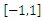with 100 nodes
Finally, let us consider the triangular function defined as follows: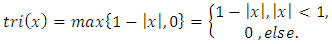On the other hand, the triangular function is defined as the convolution of the rectangular function with itself, i.e.,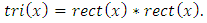Therefore,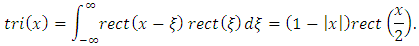Using the relation between the rectangular and Heaviside functions, it is possible to express the triangular function in terms of the Heaviside function. More specifically, since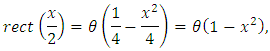by virtue of the trivial equality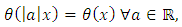then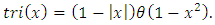The numerical comparison of the interpolation of the tri function by means of Lagrange interpolating polynomial and artificial neural networks is shown in Figures 8-10. Figure 9 shows that when we consider 50 nodes within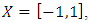the quadratic error between the Lagrange interpolating polynomial and artificial neural network interpolation dramatically decreases up to 10th epochs. From 6th epoch on, the quadratic error remains almost unaltered and is of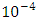order.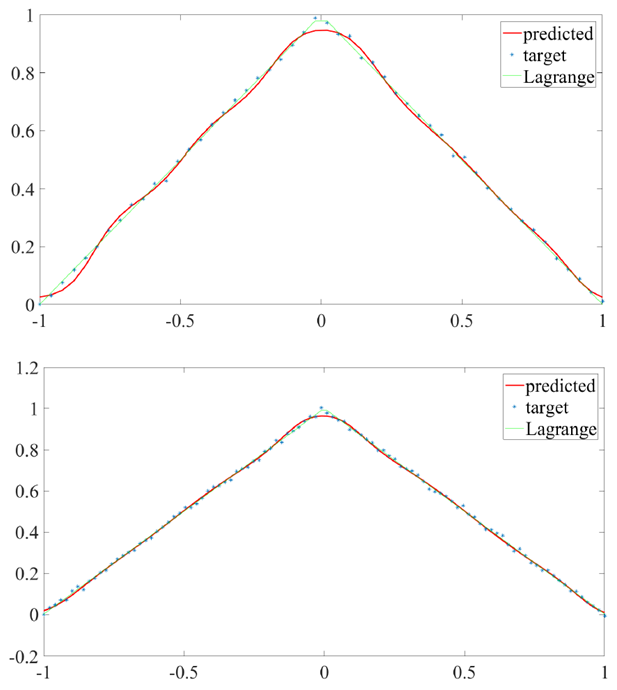Figure 8. Approximation of triangular function in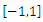with 50 nodes (upper) and 100 nodes (lower): ANN algorithm requires 12 and 30 iterations, respectively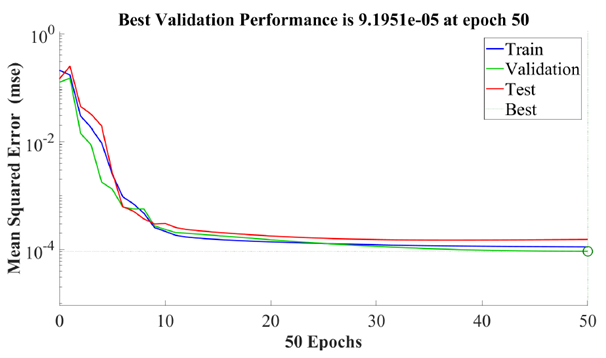Figure 9. Quadratic error approximation of triangular function in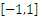with 100 nodes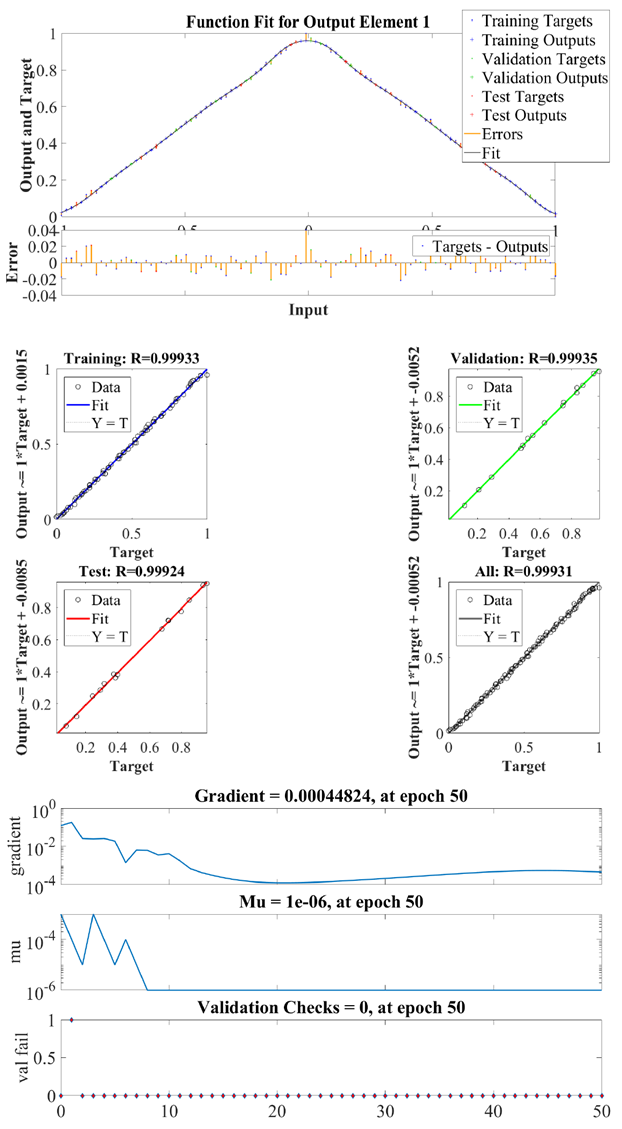Figure 10. Function fit (upper) and regression behavior (middle) and network performance (lower) for triangular function i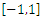with 100 nodes

### 5. Conclusions

In this paper we have studied the possibility of involving a computational neural network with a single hidden layer trained to interpolate functions with minimal number of nodes. The neural network is trained to start with the first several nodes and predict the proceeding nodes using the nearest-neighbour algorithm. The interpolation provided by the artificial neural networked has been compared numerically with the Lagrange interpolating polynomial. The algorithm is tested for the rectangular, ramp and triangular functions. It is observed that the quadratic error between the two interpolating methods dramatically decreases when the number of epochs is increased.

### Notes

1. For the sake of simplicity, we restrict ourselves by the one-dimensional case. Higher dimensional (multivariate) cases are treated in the same way.
2. Again for the sake of simplicity we consider real-valued functions. Complex-valued functions can be considered similarly.

### References

  H. Triebel (1978), Interpolation Theory, Function Spaces, Differential Operators. Elsevier Science Publishing, Amsterdam.  J. Szabados and P. Vértesi (1990), Interpolation of Functions. World Scientific, Singapore.  E. T. Sawyer (2009), Function Theory: Interpolation and Corona Problems, American Mathematical Society, Fields Institute monographs.  A. P. P. Kumar, Greedy algorithm based deep learning strategy for user behavior prediction and decision making support. Accepted manuscript.  Broomhead D. S., Lowe D. (1988) Multivariable functional interpolation and adaptive networks // Complex Systems, vol. 2, pp. 321-335.  Cybenko G. (1989), Approximations by superpositions of sigmoidal functions //Mathematics of Control, Signals, and Systems, vol. 2, issue 4, pp. 303-314.  Hornik K. (1991), Approximation Capabilities of Multilayer Feedforward Networks // Neural Networks, vol. 4, issue 2.  Park J., Sandberg I. (1991) Universal approximation using radial basis function networks // Neural Computation, vol. 3, issue 2, pp. 246-257.  Q. Zhang, A. Benveniste (1991), Approximation by Nonlinear Wavelet Networks // IEEE Transactions on Neural Networks, vol. 6, issue 10, pp. 3417-3420.  W. Ting, Y. Sugai (1999), Wavelet Neural Network for the Approximation of Nonlinear Multivariable Function // IEEE Transactions on Neural Networks, vol. 14, pp. 378-383.  Snell E. S., Gopal S., R. K. Kaufmann (2000), Spatial interpolation of surface air temperatures using artificial neural networks: Evaluating their use for down-scaling GCMs // Journal of Climate, vol. 13, pp. 886-895.  J. P. Rigol, C. H. Jarvis, N. Stuart (2001), Artificial neural networks as a spatial interpolation // International Journal of Geographical Information Science, vol.15, issue 4, pp. 323-343.  Zhang W., Barrion A. (2006), Function Approximation and Documentation of Sampling Data Using Artificial Neural Networks // Environmental Monitoring and Assessment, 122: 185. https://doi.org/10.1007/s10661-005-9173-6.  Zainuddin Z., Ong P. (2007), Function approximation using artificial neural networks // WSEAS Transactions on Mathematics, vol. 1, issue 4, pp. 173-178.  Bhaskaran P., Kumar R. R., Barman R., Muthalagu R. (2010), A new approach for deriving temperature and salinity fields in the Indian ocean using artificial neural networks Journal of Marine Science and Technology, vol. 15, pp. 160-175.  V. Nevtipilova, J. Pastwa, M. S. Boori, V. Vozenilek (2014), Testing Artificial Neural Network (ANN) for Spatial Interpolation // Journal of Geology & Geophysics, doi:10.4172/2329-6755.1000145.  Ferrari S., Stengel F., Smooth Function Approximation Using Neural Networks. Lecture notes. http://ce.sharif.edu/courses/84-85/2/ce667/resources/root/Seminar_no_10/tnnlatestmanu.pdf.  R. T. Maylavarapu, B. K. Maylavarapu, H. Sargsyan (2018) Interpolation of Generalized Functions Using Artificial Neural Networks. Accepted manuscript.